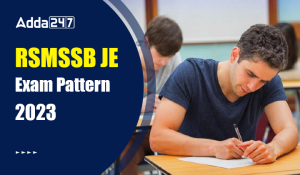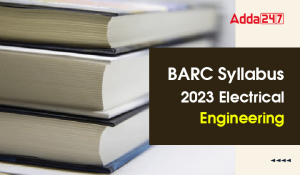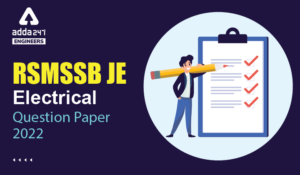Engineering Jobs   »   Quiz: Electrical Engineering 25 July 2020

# Quiz: Electrical Engineering 25 July 2020

Quiz: Electrical Engineering
Exam: RSMSSB-JEn
Topic: Miscellaneous

Each question carries 1 mark.
Negative marking: 1/3 mark
Time: 10 Minute

Q1. 100% string efficiency means
(a) self-capacitance is zero
(b) shunt capacitance is maximum
(c) self-capacitance is maximum
(d) shunt capacitance is zero

Q2. Wave-wound generators provide
(a) less current but more voltage
(b) more current but less voltage
(c) more current and more voltage
(d) none of the above

Q3. In a synchronous motor, the stator frame is made of………
(a) Stainless steel
(b) CRGO
(c) Cast iron or welded steel plates
(d) Laminated silicon steel

Q4. A 230 V, 50 Hz single-phase energy meter has a load current of 10 A at p.f. of 0.8 lagging. The energy consumed by the load in 2 minutes is
(a) 1.2 kWh
(b) 0.06 kWh
(c) 2.4 kWh
(d) 4.2 kWh

Q5. Field control of a DC shunt motor gives–
(a) Constant kW drive
(b) Constant torque drive
(c) Constant speed drive

Q6. One lux is:
(a) 1 lumen/m²
(b) 4π lumen/mt²
(c) 1 lumen mt
(d) 1 candela

Q7. Hard magnetic materials are used for manufacturing ……
(a)Insulators
(b)Temporary magnets
(c)Permanent magnets
(d)Conductors

Q8. If a conductor of length ‘l’ is moving with a velocity of ‘v’ in a magnetic field B. The induced emf will be
(a)B/lv
(b)Blv
(c)Bl/v
(d)l/Bv

Q9.A circuit has an impedance of (1 – j 2) Ω. The susceptance of the circuit is …….
(a) 0.1 S
(b) 0.2 S
(c) 0.4 S
(d) None of these

Q10. The relative permittivity of a material is 10. Its absolute permittivity will be
(a) 8.854×10^(-11) F/m
(b) 9×10^8 F/m
(c) 5×10^(-5) F/m
(d) 9×10^5 F/m

SOLUTIONS

S1. Ans.(d)
Sol. If shunt capacitance is zero the charging current through each disc will be the same. This means that voltage across each disc is the same. This in turn means 100% string efficiency.

S2. Ans.(a)
Sol. Wave – wound has only two parallel paths. Wave-wound generators provide less current but more voltage.

S3. Ans.(c)
Sol. in synchronous motor: –
(A)Stator frame → made up of Cast iron/welded steel plates
(B)Core → made up of silicon steel

S4. Ans.(b)
Sol. Energy consumed by the load in 2 minutes
=(V I cos⁡ϕ)/1000×t=(230 × 10 × 0.8)/1000×2/60 = 0.06 KWh

S5. Ans.(a)
Sol. There are 3 types of speed control for dc drives: –
(i) Armature voltage control
(ii) Field flux control
(iii) Armature resistance control
We know▭(N∝1/ϕ)
Armature voltage control technique gives high efficiency but below base speed.
So, above base speed, Field flux control is used, where by weakening the field, speed above base speed can be achieved because these two are inversely proportional to each other.

S6. Ans.(a)
Sol. One lux=1 lumen/m²
S7. Ans.(c)
Sol. Hard magnets, also referred to as permanent magnets, are magnetic materials that retain their magnetism after being magnetised. It is used for manufacturing permanent magnets.

S8. Ans.(b)
Sol. The emf induced in a straight conductor of length l moving with velocity v perpendicular to a magnetic field B is: E =Blvsinθ.

S9. Ans.(c)
Sol.
B=Xc/Z^2 =2/((1)^2+(2)^2 )=2/5=0.4 S

S10. Ans.(a)
Sol. εr=ε/εo
∴ Absolute permittivity, = ε=ε0 εr=8.854×10^(-12)×10=8.854×10^- 11 F/m

Sharing is caring!

### TOPICS:

•RSMSSB JE Exam Pattern 2023, Check Lates...
•BARC Syllabus 2023 Electrical Engineerin...
•RSMSSB JE 2022 DV Schedule, Download RSM...
•RSMSSB JE Result 2022, OUT-Direct Link t...
•RSMSSB Computer Instructor Exam date 202...
•RSMSSB JE Electrical Question Paper 2022...